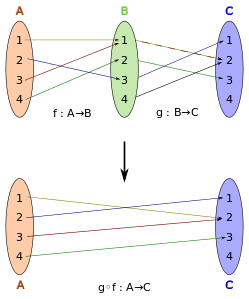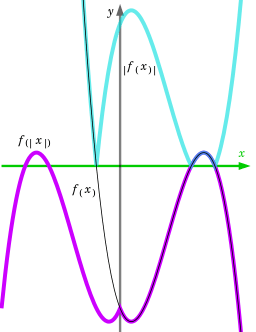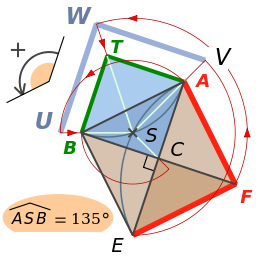# Function composition

In mathematics, function composition is the pointwise application of one function to the result of another to produce a third function. For instance, the functions f : XY and g : YZ can be composed to yield a function which maps x in X to g(f(x)) in Z. Intuitively, if z is a function of y, and y is a function of x, then z is a function of x. The resulting composite function is denoted g ∘ f : XZ, defined by (g ∘ f )(x) = g(f(x)) for all x in X.[note 1] The notation g ∘ f is read as "g circle f ", or "g round f ", or "g composed with f ", "g after f ", "g following f ", or "g of f", or "g on f ". Intuitively, composing two functions is a chaining process in which the output of the inner function becomes the input of the outer function.

The composition of functions is a special case of the composition of relations, so all properties of the latter are true of composition of functions. The composition of functions has some additional properties.

## Examplesg ∘ f, the composition of f and g. For example, (g ∘ f )(c) = #.Concrete example for the composition of two functions.
• Composition of functions on a finite set: If f = {(1, 3), (2, 1), (3, 4), (4, 6)}, and g = {(1, 5), (2, 3), (3, 4), (4, 1), (5, 3), (6, 2)}, then gf = {(1, 4), (2, 5), (3, 1), (4, 2)}.
• Composition of functions on an infinite set: If f: ℝ → ℝ (where is the set of all real numbers) is given by f(x) = 2x + 4 and g: ℝ → ℝ is given by g(x) = x3, then:
(fg)(x) = f(g(x)) = f(x3) = 2x3 + 4, and
(gf)(x) = g(f(x)) = g(2x + 4) = (2x + 4)3.
• If an airplane's elevation at time t is given by the function h(t), and the oxygen concentration at elevation x is given by the function c(x), then (ch)(t) describes the oxygen concentration around the plane at time t.

## Properties

The composition of functions is always associative—a property inherited from the composition of relations. That is, if f, g, and h are three functions with suitably chosen domains and codomains, then f ∘ (g ∘ h) = (f ∘ g) ∘ h, where the parentheses serve to indicate that composition is to be performed first for the parenthesized functions. Since there is no distinction between the choices of placement of parentheses, they may be left off without causing any ambiguity.

In a strict sense, the composition g ∘ f can be built only if f's codomain equals g's domain; in a wider sense it is sufficient that the former is a subset of the latter.[note 2] Moreover, it is often convenient to tacitly restrict f's domain such that f produces only values in g's domain; for example, the composition g ∘ f of the functions f : (−∞,+9] defined by f(x) = 9 − x2 and g : [[interval (mathematics)#Infinite endpoints|[0,+∞)]] → ℝ defined by g(x) = √x can be defined on the interval [−3,+3].Compositions of two real functions, absolute value and a cubic function, in different orders show a non-commutativity of the composition.

The functions g and f are said to commute with each other if g ∘ f = f ∘ g. Commutativity is a special property, attained only by particular functions, and often in special circumstances. For example, |x| + 3 = |x + 3| only when x ≥ 0. The picture shows another example.

The composition of one-to-one functions is always one-to-one. Similarly, the composition of two onto functions is always onto. It follows that composition of two bijections is also a bijection. The inverse function of a composition (assumed invertible) has the property that (f ∘ g)−1 = ( g−1f−1).

Derivatives of compositions involving differentiable functions can be found using the chain rule. Higher derivatives of such functions are given by Faà di Bruno's formula.

## Composition monoids

Main article: Transformation monoid

Suppose one has two (or more) functions f: XX, g: XX having the same domain and codomain; these are often called transformations. Then one can form chains of transformations composed together, such as ffgf. Such chains have the algebraic structure of a monoid, called a transformation monoid or (much more seldom) composition monoid. In general, transformation monoids can have remarkably complicated structure. One particular notable example is the de Rham curve. The set of all functions f: XX is called the full transformation semigroup or symmetric semigroup on X. (One can actually define two semigroups depending how one defines the semigroup operation as the left or right composition of functions.)The similarity that transforms triangle EFA into triangle ATB is the composition of a homothety H  and a rotation R, of which the common centre is S.  For example, the image of A  under the rotation R is U,  which may be written  R (A) = U.  And  H(U) = B  means that the mapping H transforms U  into B.  Thus  H(R (A)) = (H ∘ R )(A) = B.

If the transformation are bijective (and thus invertible), then the set of all possible combinations of these functions forms a transformation group; and one says that the group is generated by these functions. A fundamental result in group theory, Cayley's theorem, essentially says that any group is in fact just a group of permutations (up to isomorphism).

The set of all bijective functions f: XX (called permutations) forms a group with respect to the composition operator. This is the symmetric group, also sometimes called the composition group.

In the symmetric semigroup (of all transformations) one also finds a weaker, non-unique notion of inverse (called a pseudoinverse) because the symmetric semigroup is a regular semigroup.

## Functional powers

Main article: Iterated function

If Y X, then f: XY may compose with itself; this is sometimes denoted as f 2. That is:

(ff)(x) = f(f(x)) = f2(x)
(fff)(x) = f(f(f(x))) = f3(x)

More generally, for any natural number n ≥ 2, the nth functional power can be defined inductively by fn = ffn−1 = fn−1f. Repeated composition of such a function with itself is called iterated function.

• By convention, f0 is defined as the identity map on f's domain, idX.
• If even Y = X and f: XX admits an inverse function f−1, negative functional powers fn are defined for n > 0 as the opposite power of the inverse function: fn = (f−1)n.

Note: If f takes its values in a ring (in particular for real or complex-valued f), there is a risk of confusion, as fn could also stand for the n-fold product of f, e.g. f2(x) = f(x) · f(x). For trigonometric functions, usually the latter is meant, at least for positive exponents. For example, in trigonometry, this superscript notation represents standard exponentiation when used with trigonometric functions: sin2(x) = sin(x) · sin(x). However, for negative exponents (especially 1), it nevertheless usually refers to the inverse function, e.g., tan−1 = arctan ≠ 1/tan.

In some cases, when, for a given function f, the equation gg = f has a unique solution g, that function can be defined as the functional square root of f, then written as g = f1/2.

More generally, when gn = f has a unique solution for some natural number n > 0, then fm/n can be defined as gm.

Under additional restrictions, this idea can be generalized so that the iteration count becomes a continuous parameter; in this case, such a system is called a flow, specified through solutions of Schröder's equation. Iterated functions and flows occur naturally in the study of fractals and dynamical systems.

To avoid ambiguity, some mathematicians choose to write f °n for the n-th iterate of the function f.

## Alternative notations

Many mathematicians, particularly in group theory, omit the composition symbol, writing gf for gf.

In the mid-20th century, some mathematicians decided that writing "gf" to mean "first apply f, then apply g" was too confusing and decided to change notations. They write "xf" for "f(x)" and "(xf)g" for "g(f(x))". This can be more natural and seem simpler than writing functions on the left in some areas – in linear algebra, for instance, when x is a row vector and f and g denote matrices and the composition is by matrix multiplication. This alternative notation is called postfix notation. The order is important because matrix multiplication is non-commutative. Successive transformations applying and composing to the right agrees with the left-to-right reading sequence.

Mathematicians who use postfix notation may write "fg", meaning first apply f and then apply g, in keeping with the order the symbols occur in postfix notation, thus making the notation "fg" ambiguous. Computer scientists may write "f ; g" for this, thereby disambiguating the order of composition. To distinguish the left composition operator from a text semicolon, in the Z notation the character is used for left relation composition. Since all functions are binary relations, it is correct to use the [fat] semicolon for function composition as well (see the article on composition of relations for further details on this notation).

## Composition operator

Main article: Composition operator

Given a function g, the composition operator Cg is defined as that operator which maps functions to functions asComposition operators are studied in the field of operator theory.

## In programming languages

Function composition appears in one form or another in numerous programming languages.

## Multivariate functions

Partial composition is possible for multivariate functions. The function resulting when some argument xi of the function f is replaced by the function g is called a composition of f and g in some computer engineering contexts, and is denoted f |xi = gWhen g is a simple constant b, composition degenerates into a (partial) valuation, whose result is also known as restriction or co-factor.In general, the composition of multivariate functions may involve several other functions as arguments, as in the definition of primitive recursive function. Given f, a n-ary function, and n m-ary functions g1, ..., gn, the composition of f with g1, ..., gn, is the m-ary function.

This is sometimes called the generalized composite of f with g1, ..., gn. The partial composition in only one argument mentioned previously can be instantiated from this more general scheme by setting all argument functions except one to be suitably chosen projection functions. Note also that g1, ..., gn can be seen as a single vector/tuple-valued function in this generalized scheme, in which case this is precisely the standard definition of function composition.

A set of finitary operations on some base set X is called a clone if it contains all projections and is closed under generalized composition. Note that a clone generally contains operations of various arities. The notion of commutation also finds an interesting generalization in the multivariate case; a function f of arity n is said to commute with a function g of arity m if f is a homomorphism preserving g, and vice versa i.e.:.

A unary operation always commutes with itself, but this is not necessarily the case for a binary (or higher arity) operation. A binary (or higher arity) operation that commutes with itself is called medial or entropic.

## Generalizations

Composition can be generalized to arbitrary binary relations. If RX × Y and SY × Z are two binary relations, then their composition SR is the relation defined as {(x, z) ∈ X × Z : yY. (x, y) ∈ R (y, z) ∈ S}. Considering a function as a special case of a binary relation (namely functional relations), function composition satisfies the definition for relation composition.

The composition is defined in the same way for partial functions and Cayley's theorem has its analogue called Wagner-Preston theorem.

The category of sets with functions as morphisms is the prototypical category. The axioms of a category are in fact inspired from the properties (and also the definition) of function composition. The structures given by composition are axiomatized and generalized in category theory with the concept of morphism as the category-theoretical replacement of functions. The order inversion in the formula (f ∘ g)−1 = (g−1f−1) applies for groups in general and for the inverse relation; each of these is a dagger category.

## Typography

The composition symbol ∘  is encoded as U+2218 RING OPERATOR (HTML `&#8728;`); see the Degree symbol article for similar-appearing Unicode characters. In TeX, it is written `\circ`.

## Notes

1. Some authors use f ∘ g : XZ, defined by (f ∘ g )(x) = g(f(x)) instead.
2. The strict sense is used, e.g., in category theory, where a subset relation is modelled explicitly by an inclusion function.

## References

1. Daniel J. Velleman (2006). How to Prove It: A Structured Approach. Cambridge University Press. p. 232. ISBN 978-1-139-45097-3.
2. Nancy Rodgers (2000). Learning to Reason: An Introduction to Logic, Sets, and Relations. John Wiley & Sons. pp. 359–362. ISBN 978-0-471-37122-9.
3. Christopher Hollings (2014). Mathematics across the Iron Curtain: A History of the Algebraic Theory of Semigroups. American Mathematical Society. p. 334. ISBN 978-1-4704-1493-1.
4. Pierre A. Grillet (1995). Semigroups: An Introduction to the Structure Theory. CRC Press. p. 2. ISBN 978-0-8247-9662-4.
5. Pál Dömösi; Chrystopher L. Nehaniv (2005). Algebraic Theory of Automata Networks: A Introduction. SIAM. p. 8. ISBN 978-0-89871-569-9.
6. Nathan Carter (9 April 2009). Visual Group Theory. MAA. p. 95. ISBN 978-0-88385-757-1.
7. Olexandr Ganyushkin; Volodymyr Mazorchuk (2008). Classical Finite Transformation Semigroups: An Introduction. Springer Science & Business Media. p. 24. ISBN 978-1-84800-281-4.
8. Oleg A. Ivanov (1 January 2009). Making Mathematics Come to Life: A Guide for Teachers and Students. American Mathematical Soc. pp. 217–. ISBN 978-0-8218-4808-1.
9. Jean Gallier (2011). Discrete Mathematics. Springer. p. 118. ISBN 978-1-4419-8047-2.
10. Michael Barr; Charles Wells (1998). Category Theory for Computing Science (PDF). p. 6. This is the updated and free version of book originally published by Prentice Hall in 1990 as ISBN 978-0-13-120486-7.
11. ISO/IEC 13568:2002(E), p. 23
12. Bryant, R.E. (August 1986). "Logic Minimization Algorithms for VLSI Synthesis" (PDF). IEEE Transactions on Computers. C–35 (8): 677–691. doi:10.1109/tc.1986.1676819.
13. Clifford Bergman (2011). Universal Algebra: Fundamentals and Selected Topics. CRC Press. pp. 79–80. ISBN 978-1-4398-5129-6.
14. George Tourlakis (2012). Theory of Computation. John Wiley & Sons. p. 100. ISBN 978-1-118-31533-0.
15. Clifford Bergman (2011). Universal Algebra: Fundamentals and Selected Topics. CRC Press. pp. 90–91. ISBN 978-1-4398-5129-6.
16. S. Lipscomb, "Symmetric Inverse Semigroups", AMS Mathematical Surveys and Monographs (1997), ISBN 0-8218-0627-0, p. xv
17. Peter Hilton; Yel-Chiang Wu (1989). A Course in Modern Algebra. John Wiley & Sons. p. 65. ISBN 978-0-471-50405-4.